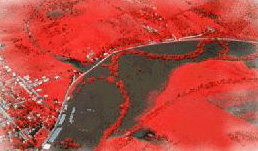Calculus on Demand at Dartmouth College Lecture 14 | Index | Lecture 16 Lecture 15## Resources

Math 3 Course Syllabus
Practice Exams

# Contents

In this lecture we take our first look at what it means to solve an equation involving derivatives. We also consider what we mean by the velocity and the acceleration of a moving object.

### Quick Question

Which is the graph of the velocity, and which the distance, for an object falling from rest near the surface of the earth?### Outline

Outlines for
Antiderivatives and Initial Value Problems
Velocity and Acceleration

### Textbook

Antiderivatives and Initial Value Problems
Velocity and Acceleration